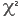# Statistical calculations on tables

You can perform a chi-squared test to see if there is a significant relationship between two variables.

You can display indexed counts or sums to give a relative measure of individual cell values.

## Applying the chi-square test

The Chi-square test can be applied to single-response variables. It compares observed (actual) and expected (theoretical) values in order to establish whether there is a significant relationship between two variables in a table.

To calculate the Chi-square Statistic and related statistics for a cross-tabulation or grid table,

• select the Chi square option in the Analysis Definition dialog
• clickon the table analysis toolbar

The Chi-square and associated statistics will be displayed above the table

You can display the expected values by selecting the Expected Counts option in the Analysis Definition dialog .These are the values that would be expected in each cell of the table if the row variable were not influenced by the column variable or vice-versa (the Null Hypothesis). They are calculated as:

(Row Total) x (Column Total) / Table Total (Base).

Since you cannot always compare Chi-squared values directly, Snap generates a statement about the result.

The interpretation of the Chi-square test result follows a seven point scale and categorises the certainty of a relationship (or non-relationship) between the variables in the cross-tabulation as follows:

The information given in the Chi-square report is as follows:

## Indexed Values

Indexed Values are widely used in product research and other projects to give a relative measure of individual cell values. Indexed Values can be shown on the table by checking the appropriate box (Indexed Counts or Indexed Sums) option in Definition tab of the Analysis Definition dialog.

In general, for any individual cell in the table, the corresponding index value can be calculated from the formula:

The figure of 100 represents a norm or expected value. Thus index values less than 100 would be considered lower than expected and index values of greater than 100 would be considered higher than expected.

Contents